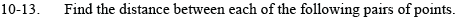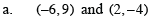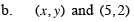Home > CCA2 > Chapter 10 > Lesson 10.1.1 > Problem10-13

10-13.
1. Find the distance between each of the following pairs of points. Homework Help ✎

1. (−6, 9) and (2, −4)

2. (x, y) and (5, 2)$\text{The distance formula is } d=\sqrt{\left(x_2-x_1\right)^2+\left(y_2-y_1\right)^2}$$d=\sqrt{\left(x-5\right)^2+\left(y-2\right)^2}$